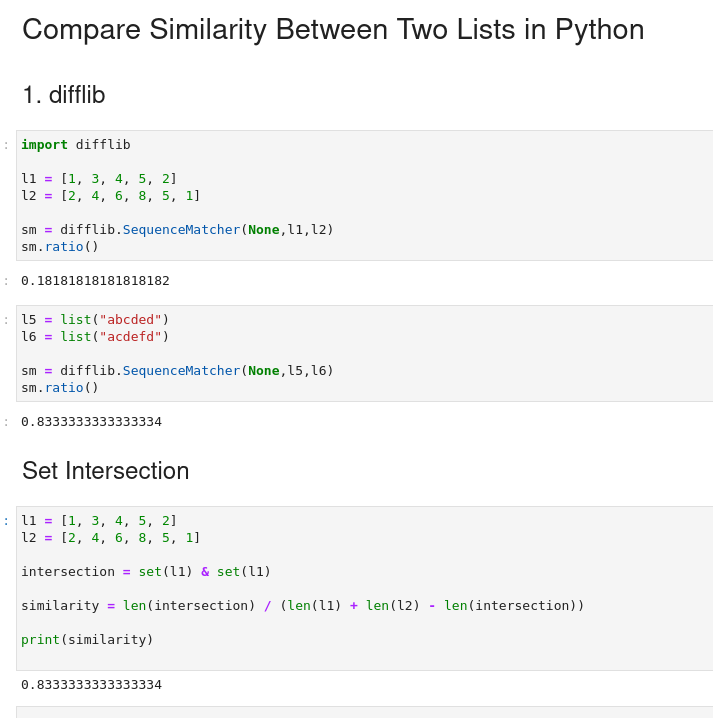To compare similarity between two lists in Python we can calculate:

• set intersection
• cosine similarity
• etc

Similarity would depend also on the data types of the items. For example:

• integer
• float
• `5.04` vs `5.03`
• string
• `grapefruit` vs `grape`

Let's cover several cases on how to compute similarity between two Python lists or arrays.

We will answer on next questions:

• python get list difference
• similarity of two lists in python
• python - find similarity between two lists
• find similarity between two words in python

## 1. Calculate similarity with difflib

Quick and efficient way to compute similarity of two numeric or string lists is done by: `difflib.SequenceMatcher(None,s1,s2)`:

### Similarity of numeric lists

``````import difflib

l1 = [1, 3, 4, 5, 2]
l2 = [2, 4, 6, 8, 5, 1]

sm = difflib.SequenceMatcher(None,l1,l2)
sm.ratio()
``````

which give use:

``````0.18181818181818182
``````

### How does it work?

While testing with:

``````l3 = [1, 3, 4, 5]
l4 = [2, 3, 4, 5]

sm = difflib.SequenceMatcher(None,l3,l4)
sm.ratio()
``````

result:

``````0.75
``````

So it seems that it compares element by element and checks if items match.

Return a measure of the sequences’ similarity as a float in the range [0, 1].

### Similarity two string lists

To find similarity of lists of words we can use the same method:

``````l5 = list("abcded")
l6 = list("acdefd")

sm = difflib.SequenceMatcher(None,l5,l6)
sm.ratio()
``````

So for lists:

• `['a', 'b', 'c', 'd', 'e', 'd']`
• `['a', 'c', 'd', 'e', 'f', 'd']`

we get:

``````0.8333333333333334
``````## 2. Set Intersection

We can find the similarity of elements of lists by checking the intersection of their elements. This is helpful when the location of the element doesn't matter:

``````l1 = [1, 3, 4, 5, 2]
l2 = [2, 4, 6, 8, 5, 1]

intersection = set(l1) & set(l1)

similarity = len(intersection) / (len(l1) + len(l2) - len(intersection))

print(similarity)
``````
• first we find the intersection
• `{1, 2, 3, 4, 5}`
• then we calculate ratio intersection length and sum of list length
• `0.8333333333333334`
• we can check also elements not present in the lists:
• `set(l1) - intersection` - elements of l1 not present in l2
• `set()`
• `set(l2) - intersection` -elements of l2 not present in l1
• `{6, 8}`

## 3. Jaccard index in Python

The Jaccard index(Jaccard similarity coefficient), is a statistical method used for finding the similarity and diversity of sample sets.

We can use Jaccard index to calculate similarity of two lists in Python:

``````l1 = [1, 3, 4, 5, 2]
l2 = [2, 4, 6, 8, 5, 1]

intersection = len(set(l1) & set(l2))
union = len(set(l1) | set(l2))

similarity = intersection / union

print(similarity)
``````

result:

``````0.5714285714285714
``````

## 5. Cosine Similarity in Python

In data science, cosine similarity helps to measure similarity between two non-zero arrays/vectors.

To calculate cosine similarity in Python we can:

``````from math import sqrt

l1 = [1, 3, 4, 5, 2]
l2 = [2, 4, 6, 8, 5, 1]

dot_product = sum(i * j for i, j in zip(l1, l2))
magnitude1 = sqrt(sum(i ** 2 for i in l1))
magnitude2 = sqrt(sum(j ** 2 for j in l2))

similarity = dot_product / (magnitude1 * magnitude2)

print(similarity)
``````

result:

``````0.9820303262342949
``````

## 6. Euclidean Distance - compare lists in Python

In mathematics, the Euclidean distance between two points in Euclidean space is the length of a line segment between the two points.

We can implement euclidean distance in Python to compare two lists:

``````import math

l1 = [1, 3, 4, 5, 2]
l2 = [2, 4, 6, 8, 5, 1]

squared_distance = sum([(i - j) ** 2 for i, j in zip(l1, l2)])
distance = math.sqrt(squared_distance)

similarity = 1 / (1 + distance)

print(similarity)
``````

result:

``````0.16952084719853724
``````

## 7. Hamming distance in Python

In information theory, the Hamming distance between two strings of equal length is the number of positions at which the corresponding symbols are different.

Examples:

• "karolin" and "kathrin" - 3
• "karolin" and "kerstin" - 3
• "kathrin" and "kerstin" - 4
• 0000 and 1111 - 4
• 2173896 and 2233796 - 3

To compare two lists of strings or numbers and find similarity we can do:

``````list1 = [1, 2, 3, 4, 5]
list2 = [2, 4, 6, 8]

distance = sum(i != j for i, j in zip(list1, list2))

similarity = 1 - (distance / len(list1))

print(similarity)
``````

Similarity of the both lists by comparing with Hamming distance is:

``````0.0
``````

we can measure hamming distance also by using module - `scipy` - but arrays should have equal size:

``````from scipy.spatial import distance

l1 = [1, 3, 4, 5, 2]
l2 = [2, 4, 6, 8, 5]

d = round(distance.hamming(l1, l2) * len(l1))
print(d)
``````

result:

``````5
``````

## Summary

In this post, we saw how to compare two lists in Python and calculate the similarity between them.

We saw detailed examples for 7 different techniques to compute similarity. The examples show the basics for comparison of sets, arrays or lists. To learn more you can read the materials in Resources.